hydraulics

# Urban Drainage Structures from Innovyze H2OCalc

3.13 Urban Drainage Structures

The methodology presented here for analysis and design of the drainage structures described in this section is based on HEC-22 manual (FHWA, 2001)

Gutter Flow

Gutters are the sections of roadway that run adjacent to the curb. Their purpose is to collect and convey surface runoff to drainage inlets and in turn to underground storm sewers. The corresponding spread of water onto the pavement, or top width of flow measured perpendicular to the edge of the roadway, is a primary concern from an analysis perspective. The lateral cross slope of a traffic lane facilitates drainage of incident rainfall to the gutter. Depending on the cross slope, conventional gutters may be grouped as uniform gutter (i.e., has uniform cross slope) or composite gutters (i.e., has multiple cross slopes).

Uniform Gutter Sections

Uniform gutters have a shallow, triangular cross section, with a curb forming the near-vertical leg of the triangle as shown in the following figure.The governing equation for uniform gutters is given aswhere Q = gutter flow rate (m3/s, ft3/s)

Kc = empirical constant (0.376 in SI, 0.56 in English)

n = Manning’s roughness coefficient

Sx = gutter cross slope (m/m, ft/ft)

SL = longitudinal slope of the road way (m/m, ft/ft)

Spread T is related to depth at the curb, d, and flow area, A, by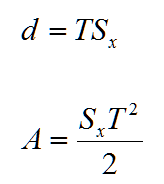Table 3-7: Manning’s n for Street and Pavement Gutters

 Type of Gutter or Pavement Manning’s n Concrete gutter, troweled finish 0.012 Asphalt Pavement: Smooth texture 0.013 Rough texture 0.016 Concrete gutter-asphalt pavement: Smooth 0.013 Rough 0.015 Concrete pavement: Float finish 0.014 Broom finish 0.016

Composite Gutter Sections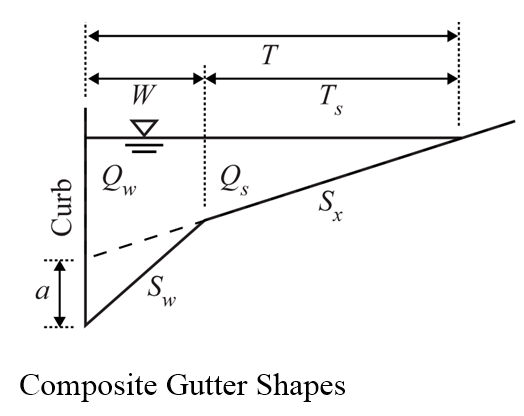Evaluation of composite gutters requires additional consideration of flow in the depressed section. The depression serves to retain more water above inlet entrances and thus increases gutter flow capacity. The relationship between total discharge, Q, and depressed gutter flow, Qw, can be expressed aswhere Qw and Qs represent portion of the gutter flows for the sections shown in the figure above (m3/s, ft3/s).

The relationship between Q and Qs is given as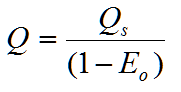where Eo = ratio of Qw to Q, or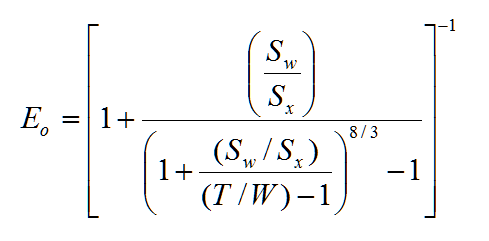(71)

where W = width of the depressed section (m, ft)

Sw = cross slope of the depressed section (m/m, ft/ft)

The slope terms and the width of depression are related through depth of the depression, a, aswhere a is the gutter depression (m, ft) illustrated in the figure given above.

Drainage Inlet

As flow accumulates in gutters and spread increases, the risk of traffic accidents and delays increases. To limit this risk, drainage inlets are needed at the edge of the roadway to intercept all or a portion of the runoff and convey it to an underground storm sewer. Although there are many types and sizes of inlets in use, they are generally classified as grate, curb-opening, combination, or slotted-drain inlets. The responsibility of the designer is to determine the type, size, and spacing of inlets that cost-effectively and safely captures runoff.

Key parameters in evaluating inlet flow conditions are capacity, Qi, and interception efficiency, E. The former refers to flow that is intercepted by a particular drainage inlet. Any gutter flow that is not intercepted is referred to as bypass, or carryover, flow, Qb. Thus, if Q is total gutter discharge,The interception efficiency of the inlet is the fraction of gutter flow that the inlet will capture under a given set of conditions, and is expressed as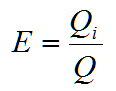Grate Inlet

A grate inlet, as shown in the figure below, consists of an opening in the gutter covered by one or more, flush-mounted grates that are placed parallel to gutter flow.Eo, defined as the ratio of frontal flow, Qw, to total gutter flow, Q, for a uniform gutter can be expressed as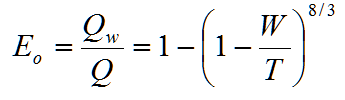where Qw = portion of flow that passes directly over the upstream side of the grate

W = width of the grade

The ratio of intercepted frontal flow to total frontal flow, also referred to as frontal flow efficiency, Rf, is defined aswhere Kf = constant (0.295 in SI, 0.09 in English)

V = gutter flow velocity (m/s, ft/s)

Vo = splash-over velocity (velocity where splash-over first occurs) (m/s, ft/s).

The following figure could be used to determine splash-over velocity based on grate type, length of the grate, and gutter flow velocity.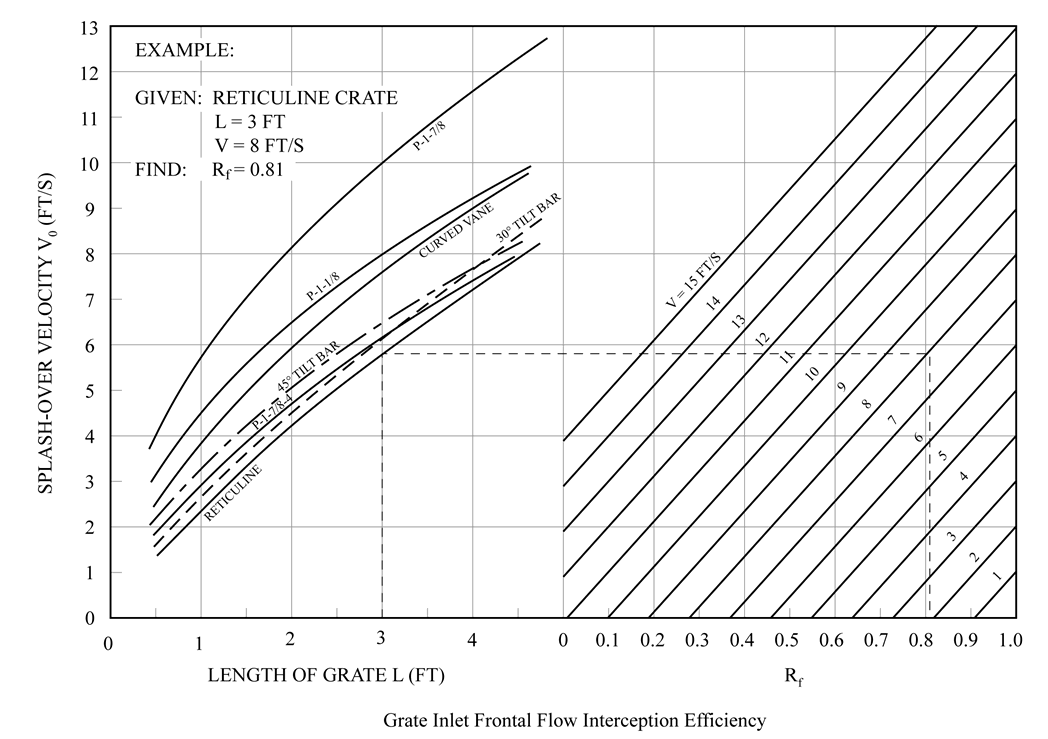Charts to Determine Slash-Over Velocity for Grate Inlets (Adapted from FHWA, 2001)

The ratio of side flow to gutter flow is expressed as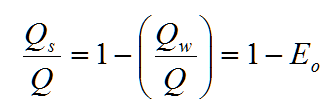where Qs = gutter flow traveling around the perimeter of the grate when spread

exceeds its width (m3/s, ft3/s)

The ratio of intercepted side flow to total side flow, referred to as side flow efficiency, Rs, is defined as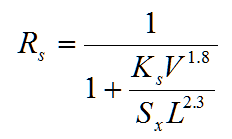where Ks = empirical constant (0.083 in SI, 0.15 in US)

L = grate length (m, ft) – The range of grate length allowed by H2OCalc is from 0.5 ft to 4.5 ft as defined in the FHWA Chart 5.

The overall inlet efficiency, E, can be evaluated from the frontal and side flow efficiencies byFrom Equation (74), the capacity of a grate inlet can be obtained by multiplying Equation (79) by the total gutter flow, or

Curb Inlet

A curb-opening inlet is comprised of a vertical opening in the curb that is covered by a concrete slab, as shown in the following figure.

For uniform cross slopes, the length of a curb-opening inlet required to intercept all gutter flow, LT (m, ft), is defined aswhere Ko = empirical constant (0.817 in SI, 0.6 in English).

The efficiency of shorter-length inlets can be evaluated bywhere L = curb-opening length (m, ft).For the case where either locally- or continuously-depressed gutter sections are used (see the following figure), Equation (82) can still be used, but an equivalent cross slope, Se, should be substituted for Sx, wherewhere Eo = ratio of Qw to Q [see Equation (75)]

Sv = cross slope of the depressed section, expressed as Sv = a/W (see the following figure).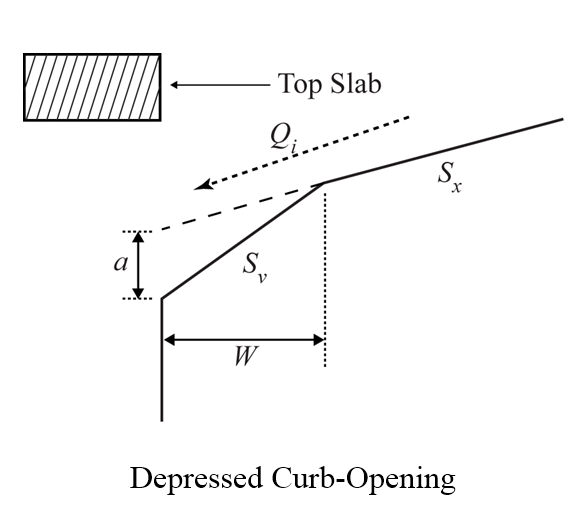Slot Inlet

Slotted drain systems, illustrated in the figure given below, are comprised of a pipe that is cut longitudinally along its crown with bars placed perpendicular to support the opening. The hydraulic characteristics of slot inlet are similar to curb opening inlets; thus, Equations (81) and (82) are applicable.Combination Inlet

Combination inlets integrate features of both curb-opening and grate inlets (see the figure below). For equal-length combination inlets, in which the grate and vertical curb opening are of the same length, inlet capacity and interception efficiency is not significantly different from that of the grate alone. Thus, Equations 75 through 80 are generally applicable.Ditch Inlet

Roadside ditches may be drained by drop inlets similar to those used for pavement drainage. The ratio of frontal flow to total flow for trapezoidal ditches (channels) is expressed as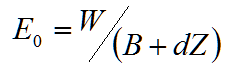where W = width of the drop inlet (m, ft)

d = depth of flow in the channel (m, ft) determined using Manning’s equation.

Z = horizontal distance of side slope to a vertical rise of 1 unit (ft, m)

Frontal flow efficiency, side flow efficiency, total efficiency, intercepted flow, and bypass flow are computed using the technique described previously for grate inlets.

Inlets in Sag

Inlets in vertical curves, or sag locations, operate as weirs at shallow ponding depths and as orifices at larger depths. At intermediate depths, flow is not well defined and may actually fluctuate between weir and orifice control. The depth at which orifice flow begins depends on grate size and bar configuration, curb opening dimensions, or the slot width of an inlet.

Grate Inlets

The capacity under weir conditions is expressed as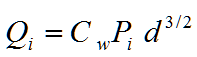where Pi = perimeter (m, ft) of the grate, not including the side adjacent to the curb, if present

Cw = weir coefficient (1.66 in SI, 3.0 in English)

d = depth at the curb (m, ft)

Under orifice conditions,where Co = orifice coefficient (0.67)

Ag = clear opening, or effective area of the grate

g = gravitational constant (9.81 m/s2, 32.2 ft/s2)

Curb inlets

Curb-opening inlets operate as weirs up to a depth (d) that is less than or equal to height (h) of the vertical opening. In this case, inlet capacity can be computed bywhere Cw = weir coefficient (1.66 in SI, 3.0 in English)

L = curb-opening length (m, ft)

If the gutter section is depressed, this relationship is modified aswhere Cw = weir coefficient (1.25 in SI, 2.3 in English)

W = lateral width of depression (m, ft)

d = throat width (m, ft) measured from the undepressed cross slope

(i.e., d = TSx)

At depths of approximately 1.4 times the opening height, curb-opening inlets tend to function as orifices. Under these conditions, capacity can be computed by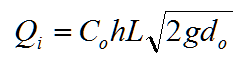where do = effective head (m, ft) (see the following three figures)

h = throat width (m, ft) (see the following three figures)(a) Horizontal Throat(b) Inclined Throat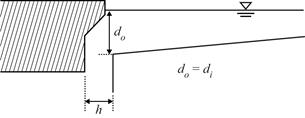(c) Vertical Throat

Throat Configuration

Slot Inlets

Due to vulnerability to clogging, slotted drain inlets are not recommended for sag locations. Nevertheless, if they exist, slotted drains operate as weirs when depth at the slot is below approximately 0.2 ft (60 mm) and as orifices for depths greater than 0.4 ft (120 mm). Capacity of a slotted drain operating under weir conditions can be evaluated using Equation (87). The corresponding weir coefficient varies with flow depth and slot length, but a typical value is 1.4 in SI and 2.48 in English. Under orifice conditions, capacity is a function of the slot width, W, and is computed byCombination Inlets

Combination inlets, especially those of a sweeper configuration, can be effective in sag locations. In this case, the sweeper inlet can extend from both sides of the grate. Similar to on-grade computations, under weir conditions, the inlet capacity of an equal-length combination inlet is essentially equal to that of the grate alone. Under orifice conditions, the capacity increases to the sum of that of the grate (i.e., Equation 86) and that of the curb opening (i.e., Equation 89). For a conservative design, however, it is recommended that combination inlet be designed assuming complete clogging of the grate.

Ditch Inlets

Ditch inlets in sag intercept 100 % of the gutter flow.